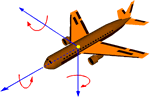#### You may also like### Roots and Coefficients

If xyz = 1 and x+y+z =1/x + 1/y + 1/z show that at least one of these numbers must be 1. Now for the complexity! When are the other numbers real and when are they complex?### Target Six

Show that x = 1 is a solution of the equation x^(3/2) - 8x^(-3/2) = 7 and find all other solutions.### 8 Methods for Three by One

This problem in geometry has been solved in no less than EIGHT ways by a pair of students. How would you solve it? How many of their solutions can you follow? How are they the same or different? Which do you like best?

# Complex Rotations

##### Age 16 to 18 Challenge Level:If you have solved quadratic equations you have met complex numbers. For example if you solve the equation $$x^2-4x+13=0$$ you get the solutions $x=2\pm\sqrt{-9}=2\pm3i$ where $i=\sqrt{-1}$.

The complex number $a+ib$ is represented in the plane by the point with coordinates $(a,b)$. This is called an Argand diagram. Make your own choice of some complex numbers, and mark them on a graph with lines joining the points to the origin. Now multiply your numbers by $-1$ and join their images to the origin. Make and prove a conjecture about the geometric effect of multiplying complex numbers by $-1$.

Again make a choice of some complex numbers and multiply each one by $i$. Draw the complex numbers and their images on a graph and make and prove a conjecture about the effect of multiplying complex numbers by $i$.

What happens if you multiply a complex number by $i$ twice, three times, four times, ..., $n$ times?